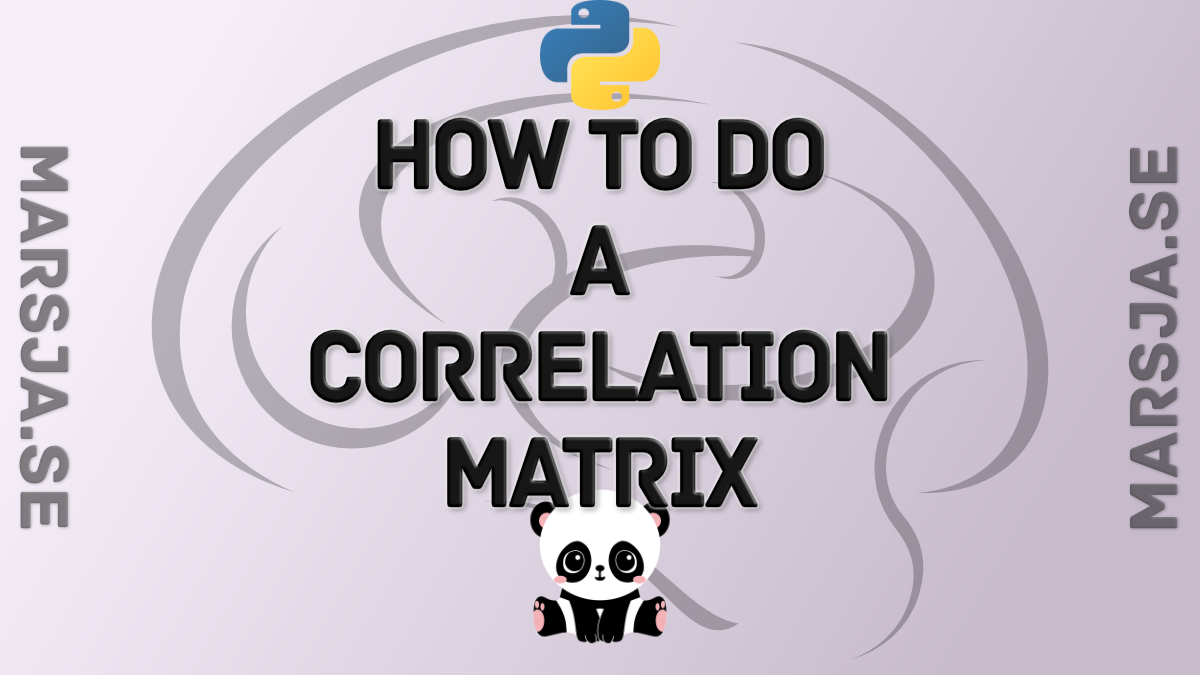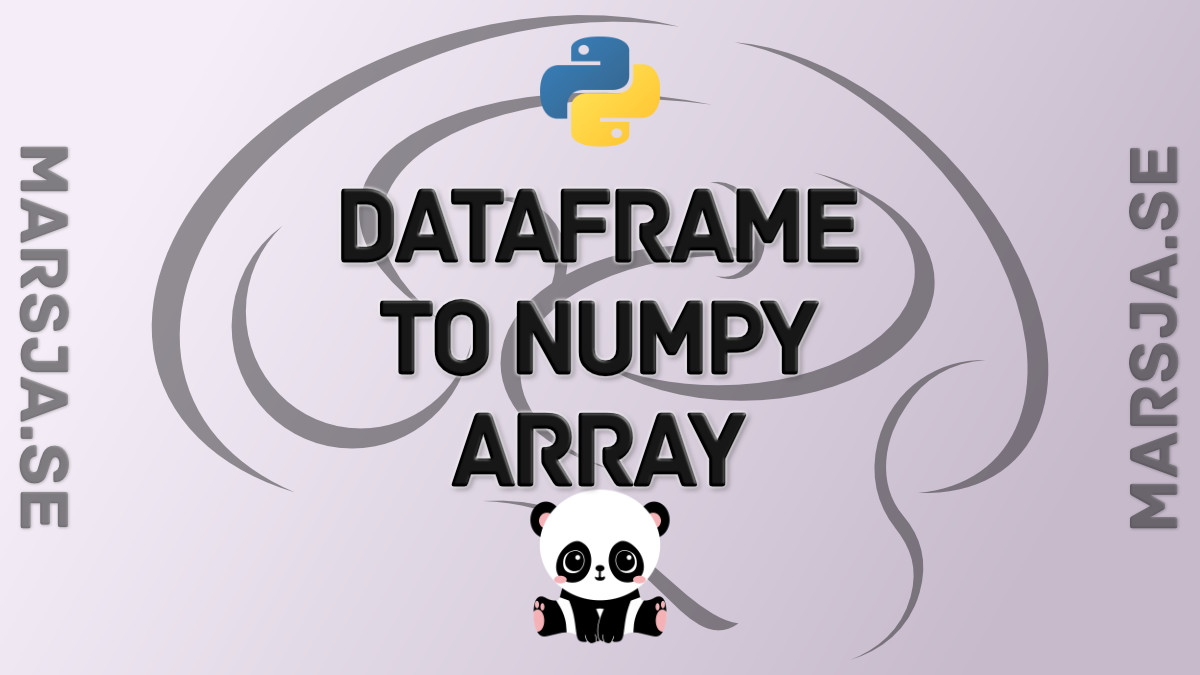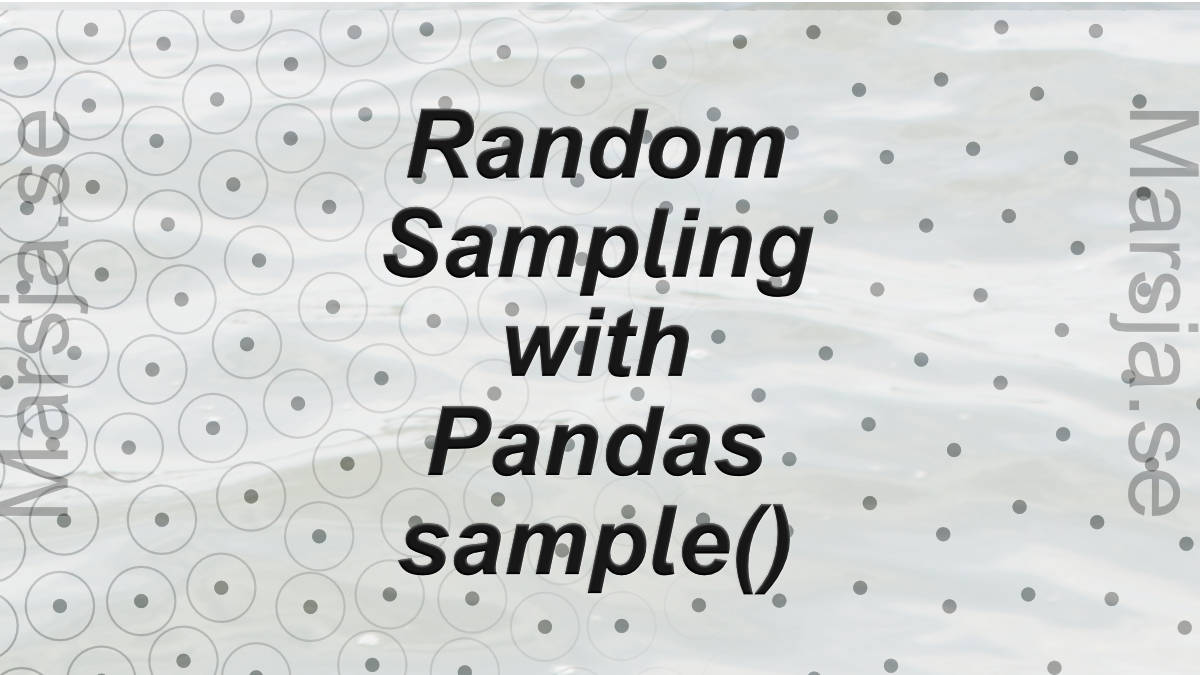## Create a Correlation Matrix in Python with NumPy and Pandas

In this post, we will go through how to calculate a correlation matrix in Python with NumPy and Pandas. Now, there will be a number of Python correlation matrix examples in this tutorial. First, we will read data from a CSV fil so we can, in a simple way, have a look...## How to Convert a Pandas DataFrame to a NumPy Array

In this short Python Pandas tutorial, we will learn how to convert a Pandas dataframe to a NumPy array.  Specifically, we will learn how easy it is to transform a dataframe to an array using the two methods values and to_numpy, respectively. Furthermore, we will...## The Easiest Data Cleaning Method using Python & Pandas

In this post, we are going to learn how to do simplify our data preprocessing work using the Python package Pyjanitor. More specifically, we are going to learn how to: Add a column to a Pandas dataframeRemove missing valuesRemove an empty columnCleaning up column...## How to use Pandas Sample to Select Rows and Columns

In this tutorial, we will learn how to use Pandas sample to randomly select rows and columns from a Pandas dataframe. There are some reasons for randomly sample our data; for instance, we may have a very large dataset and want to build our models on a smaller sample...

## Step-by-step guide for solving the Pyvttbl Float and NoneType error

In this short post I will show you a quick fix for the error “unsupported operand type(s) for +: ‘float’ and ‘NoneType’” with Pyvttbl. In earlier posts I have showed how to carry out ANOVA using Pyvttbl (among other packages. See posts 1, 2, 3, and 4 for ANOVA...